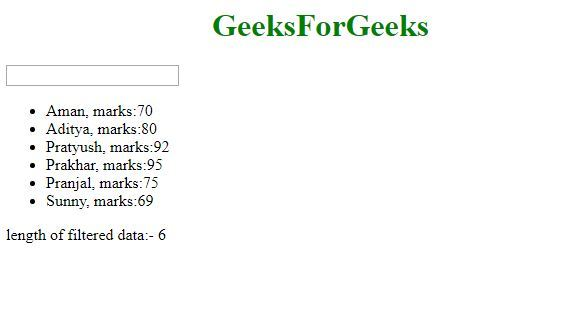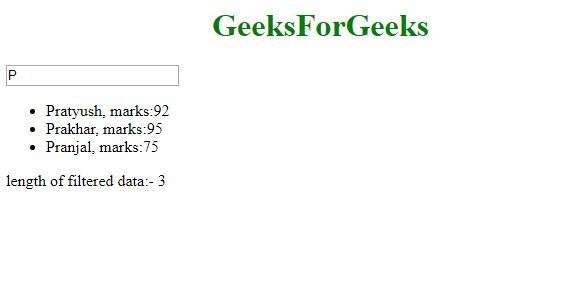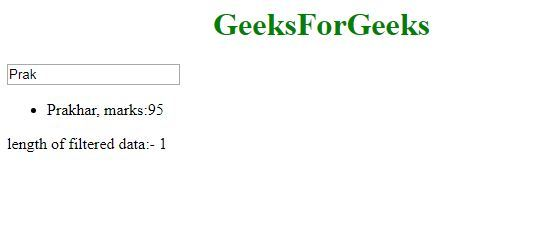GeeksforGeeks App
Open AppBrowser
Continue

# How to display length of filtered ng-repeat data in AngularJS ?

The task is to Display the length of filtered ng-repeat data. Here we are going to use alias expression to solve this problem.

Approach: To display the length of filtered ng-repeat data we use an alias expression. We create an alias for the variable used to filter the ng-repeat data and display the length of the alias at each moment.

Syntax:

```<element ng-repeat="expression | filter: search as result"> Content... </element>
<p> {{ result.length }} <p>
```

Example:

 ```<``html``>``     ` `<``head``>``    ``<``title``>``        ``Angular JS Filtered data length``    ````     ` `    ``<``script` `src` `= ``"https://ajax.googleapis.com/ajax/libs/angularjs/1.3.14/angular.min.js"``>``    ``````     ` `<``body` `> ``    ``<``h1` `style` `= ``"color:green; text-align:center;"` `> ``        ``GeeksForGeeks ``    `` ``     ` `    ``<``div` `ng-app` `= ``"mainApp"` `ng-controller` `= ``"studentController"``>``        ``<``input` `type` `= ``"text"` `ng-model` `= ``"searchName"``>``            ``<``br``/>``         ` `        ``<``ul``>``            ``<``li` `ng-repeat` `= ``                ``"student in students | filter: searchName as result"``>``                ``{{ student.name + ', marks:' + student.marks }}``            ````        ````        ``<``p``>length of filtered data:- {{ result.length }}``    ````     ` `    ``<``script``>``        ``var mainApp = angular.module("mainApp", []);``         ` `        ``mainApp.controller('studentController', function(\$scope) {``            ``\$scope.students = [``                ``{name:'Aman', marks:70},``                ``{name:'Aditya', marks:80},``                ``{name:'Pratyush', marks:92},``                ``{name:'Prakhar', marks:95},``                ``{name:'Pranjal', marks:75},``                ``{name:'Sunny', marks:69}``            ``];``        ``});``    `````` ` ``

Explanation:
Here, we are displaying the details of students and there is a search box to search by name of student. Now, we bind the input of that search box in AngularJS searchName variable. SearchName variable is used to filter, we create an alias of it which is result here and then we display the result.length. So as the length of filtered ng-repeat data change, length of result also change.

Output:
Initial screen would be:Then after the search, filtered ng-repeat data length change:My Personal Notes arrow_drop_up
Related Tutorials